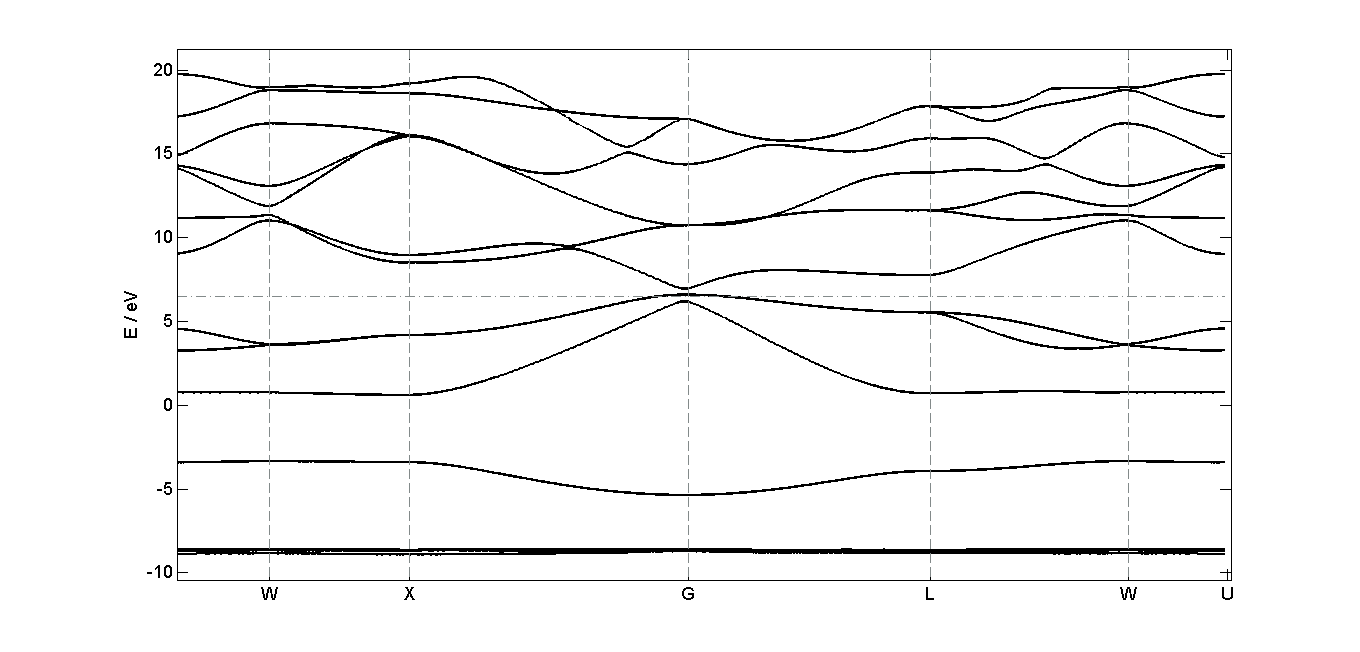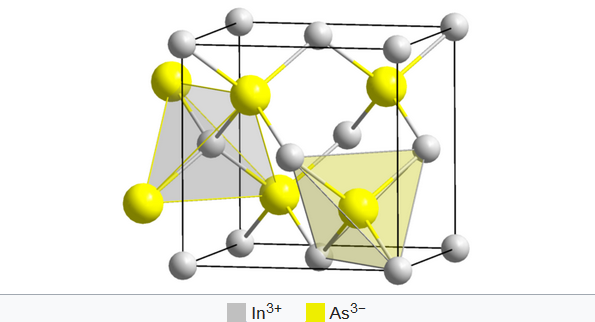## Bandstructure of indium arsenide (InAs)

The electron bandstructure for indium arsenide (zincblende structure) was calculated using the program Quantum Espresso (version 5.3.0). InAs is a compound semiconductor material with a direct bandgap of 0.35 eV. The calculated bandgap was too small, so the bands above the valence band were shifted to get the right gap. The course of the calculated bands correlates to comparative bandstructures for InAs (cf. MaterialsDesign) .Annotation:
The outcoming data of Quantum Espresso were prepared by a Matlab-Script. The script allows to choose a order of symmetry points and plots the corresponding bandstructure. Also the density of states can be plotted.

Matlab-Script:
Input files:

Output files:
InAs_data.zipZincblende structure of InAs Source: Wikipedia

Density of states